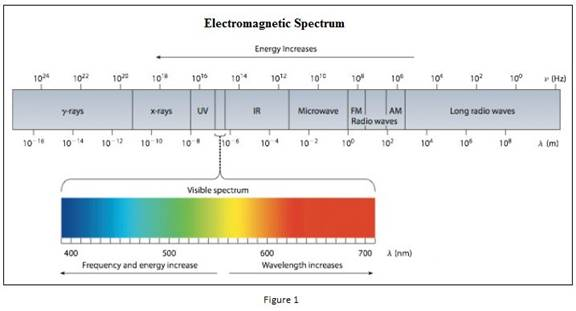Chapter 6, Problem 66GQ

Chapter
Section
Textbook Problem

The most prominent line in the emission spectrum of chromium is found at 425.4 nm. Other lines in the chromium spectrum are found at 357.9 nm, 359.3 nm, 360.5 nm, 427.5 nm, 429.0 nm, and 520.8 nm. (a) Which of these lines represents the most energetic light? (b) What color is light of wavelength 425.4 nm?

(a)

Interpretation Introduction

Interpretation: The most energetic line among the given lines in the emission spectrum of chromium has to be identified.

Concept introduction:

• Planck’s equation,

E==hcλwhere, E=energyh=Planck'sconstantν=frequency

The energy increases as the wavelength of the light decrease. Also the energy increases as the frequency of the light increases.

• The frequency of the light is inversely proportional to its wavelength.

ν=cλwhere, c=speedoflightν=frequencyλ=wavelength

Explanation

Emission spectrum of chromium has five lines in 357.9nm, 359.3nm, 360.5nm, 425.4nm, 427.5nm, 429.0nm and 520.8nm where 425.4nm is prominent

(b)

Interpretation Introduction

Interpretation: The colour of line having wavelength 425.4nm has to be identified.

Concept introduction:

• Electromagnetic radiations are a type of energy surrounding us. They are of different types like radio waves, IR, UV, X-ray etc.
• Visible light lies in the wavelength of 400nm to 700nmStill sussing out bartleby?

Check out a sample textbook solution.

See a sample solution

The Solution to Your Study Problems

Bartleby provides explanations to thousands of textbook problems written by our experts, many with advanced degrees!

Get Started

What is the hybridization of nitrogen in

Chemistry: Principles and Reactions

How does the DNA molecule produce a copy of itself?

Foundations of Astronomy (MindTap Course List)

If a suspended object A is attracted to a charged object B, can we conclude that A is charged? Explain.

Physics for Scientists and Engineers, Technology Update (No access codes included)

What is the deep scattering layer?

Oceanography: An Invitation To Marine Science, Loose-leaf Versin